•
•
•
•
•
•
•
•

# NUMBER SYSTEM SHORTCUTS AND TRICKS

Today we are going to talk about some important arithmetic formulae in the chapter “number system”. Which are very useful to all types of competitive examinations. Readers are advised to learn all the formulae and keep practicing with them.

SOME IMPORTANT SUTRAS

1). Dividend =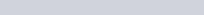2). Quotient =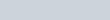3). Divisor =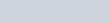4). Remainder =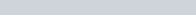5). Sum of n consecutive natural number=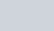6). Sum of squares of n even numbers =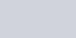7). Sum of multiples of x till to n =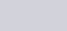8). Sum of squares of n odd numbers=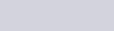9). Sum of squares of consecutive natural numbers till n =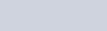10). Sum of cubes of consecutive natural numbers till n =11). Sum of consecutive even numbers till n =12). Sum of consecutive n odd numbers =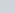13). Sum of consecutive odd numbers till n =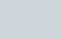14). Sum of n consecutive even numbers=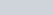15). If any number is multiplied by any other number x and in the obtained results, when x is added then it is divisible by any other number y, then the smallest divisible number = [x(y-1)+x]
16). If any number is multiplied by any other number x and in the obtained results, when x is substracted then it is divisible by any other number y, then the smallest divisible number = [x(y+1)-x]
17). If x is the sum of a number of two-digit and the number obtained by interchanging their digit, then the sum of the digit is = x/11
18). If y is the difference of a number of two-digit and the number obtained by interchanging their digit, then the difference of the digit is = y/9
19). m the sum of the digit of any two-digit number, if their digit is interchanged then the number becomes smaller by n from the original number. Then the original number(x,y) is =
x = m -[1/2({9m-n}/9)] , y =1/2({9m-n}/9)
*. If number become greater by n then the original number (x,y) =
x = m -[1/2({9m+n}/9)] , y =1/2({9m+n}/9)
20). If the sum of two numbers is x and their differences is y then their products is=(x^2-y^2)/4
21). If the sum of the number and its square is x then the number is = [√(1+4x) – 1]/2
22). If x% of a number is n, then y% or z% of that number is = (yzn/x)×100
23). If the ratio of sum and difference of two numbers is a:b, then the ratio of that two numbers = a+b/a-b
24). If the difference of the original number and the number obtained by interchanging their digit is x,  then the difference of digit is = x/9
25). If the ratio between the two-digit number and the sum of their digit is a:b if unit place digit is n more than the digit in that of tens place. Then the number is given by = (9a/11b-2a)n
*. Unit place digit = (10b-a/11b-2a)n
*. Tens place digit = (a-b/11b-2a)n
26). If f(x) = x^n +K , is divided by x-1 , then the remainder is =
*. Remainder = K+1, if K<(x-1)
*. Remainder = (1+ remainder obtained when K is divided by x-1) , if K>(x-1)
27). When a number is divided by x1 and x2 separately, it leaves remainder r1 and r2 respectively. If that number is multiplied by x1×x2, then the remainder is = (x1×r2 + r1)
28). The numbers of zeros at the end of the product is given by;
*. Number of zeros = 2^m×5^n if m>n
*. Number of zeros = m if m<n
29). When a number is multiplied by x, then it increased by y, then the number is = y/(x-1)
30). If N is a composite number and it can be expressed as N = a^p.b^q.c^r…., where a, b and C are different prime numbers and p,q and r are positive integers, then a number of divisors are =  (p+1). (q+1). (r+1)…
31). If a number is divided by N1 then it leaves remainder R, if the same number is divided by N2, then the remainder is = R/N2,
[ Note: N1>N2, and N1 is divisible by N2]
32). If the sum of two numbers is x and their differences is y, then the difference in their squares is = xy
33). The difference of the squares of two consecutive numbers X and Y are = X+ Y
34). If the sum of two numbers is X and the sum of their squares is Y, then
*. The product of the numbers = X^2-Y/2
*. The numbers are = [X-√(2Y-X^2)]/2 and        [X +√(2Y-X^2)]/2
35). If the sum of the squares of two numbers is x and the squares of their differences is y, then the product of that two numbers is = (x-y)/2
36). If the product of two numbers is x and the sum of their squares is y, then the sum of the two numbers is √(y+2x) and the difference of two numbers is √(y-2x).
37). If the denominator of a rational number is d more than its numerator. If the numerator is increased by x, and the denominator is decreased by y. Then we obtained P. Then the rational number is =
[x-P(d-y)/x+(yP-d)]
38). When A is added to another number B then total (A+B) becomes p% of number B. Then the ratio between A and B is =
(p-100/100)
39). When the two different number is divided by the same divisor, they leave remainder x and y respectively. If their sum is divided by the same divisor then the remainder is z, then the divisor is = (x+y-z)
40). If the product of two numbers is x and their sum is y, then the two numbers are =
[y+√(y^2-4x)]/2 and   [y-√(y^2-4x)]/2
41). If the product of two numbers is x and their differences is y, then the two numbers are = √(y^2+4x)+y/2 and  √(y^2+4x)-y/2